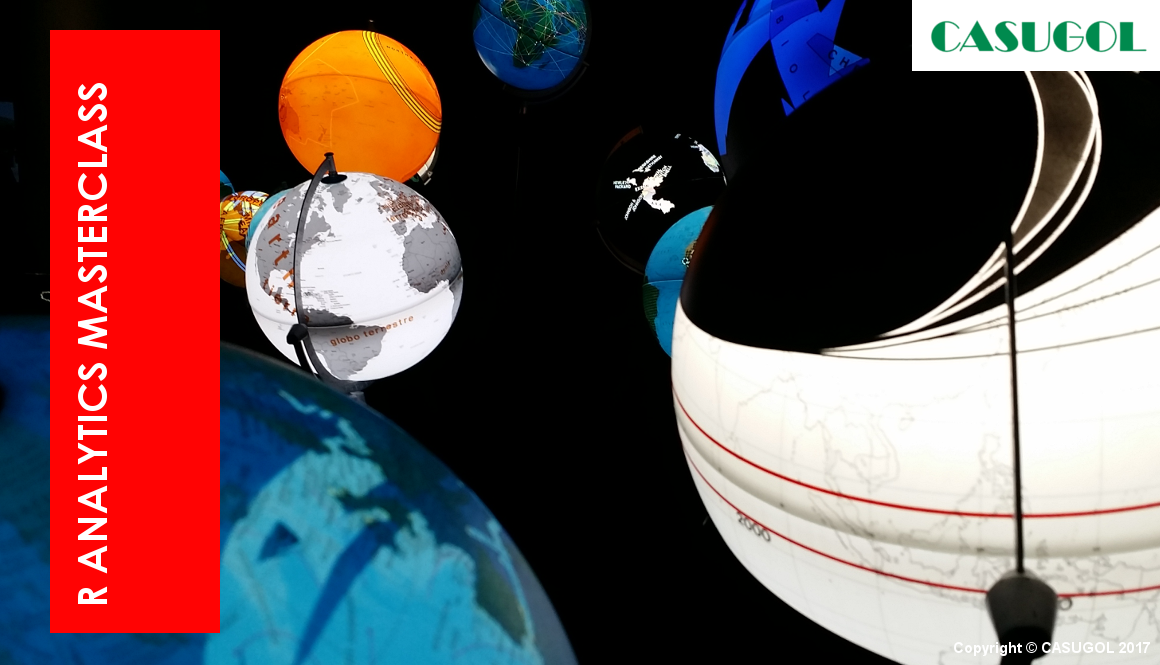# R Analytics MasterClassR is a programming language incorporated with a software environment used for statistical computing and graphics. It is capable of conducting numerical computations through additional packages. Being open source has been beneficial to organizations that specialize in research and development, or are cautious about intellectual property and data governance.

R is also used by a growing number of data analysts inside corporations and academia. It is becoming their lingua franca partly because data mining has entered a golden age, whether being used to set ad prices, find new drugs more quickly or fine-tune financial models. Companies as diverse as Google, Pfizer, Merck, Bank of America, the InterContinental Hotels Group and Shell use it.R Analytics MasterClass 17 July 2017 to 19 July 2017 Singapore, Singapore

Course Duration: 3 Days

##### COURSE OBJECTIVE

To acquire the knowledge and develop a robust set of R Analytics / Programming skills required for designing and implementation.

##### COURSE OUTLINE
• Introduction to Business Analytics & R Programming
• Why use R Programming?
• Introduction to Simple Data Visualization Charts
• How to visualize data using simple charts
• Group discussion on “How does your organization Make use of Simple Charts”
• Introduction to Data Summarization Techniques
• Types of Data Summarization Techniques
• Group discussion: How does your organization
Use simple statistics and data summarization techniques?”
• Hands-on Practice Example on Data Visualization & Summarization
using R
• Using R Programming, a business case study example is used to
visualize the data to gain business insights
• Using R Programming, a business case study is used to summarize
• Introduction to Correlation Analysis
• Business Case Study example of variables related to each other
• Group Discussion on “Which business variables in your
organization/industry can you test whether they are related or not?”
• Introduction to Statistical Significance Testing
• What is Statistical Significance
• Types of Statistical Error
• Are these two variables Related?
• How to determine if two variables are related to each other Security Management
• Some examples of business variables that are related to each other
• Hands-on Practice Example on Correlation Analysis using R
• Using R Programming, a business case study example is used as a
demonstration to determine whether two variables are related to each
other
• A Practice exercise on determining whether two variables are related
to each other.
• Introduction to Linear Regression Models
• Business case study examples on the Value of Simple Linear
Regression Models
• Group Discussion on, “Give an example of a situation in your
industry where Linear Regression Modelling may be applied”
Types of Modelling Techniques
• Types of Modelling Techniques
• Forward, Backward & Mixed Modelling Techniques
• The Goodness of Fit Measure
• Hands-on Practical Example on the Application of the Linear
Regression Model Development
• Demonstration on how to build a Linear Regression Model
• Practice exercise on how to build a Linear Regression Model
• The Linear Regression Modelling Method in Action
• The Assumption of the Errors
• The Evaluation of the Model
• Group Discussion
• Summary & Wrap-up
• R
##### WHO WILL BENEFIT FROM THIS COURSE?

This course is designed to assist those people interested in the areas of Business Analytics / R Analytics. The R Analytics MasterClass is intended to equip you with a set of skills that you can draw on to implement the technology in your organisation.

Class is limited to 20 attendees as hands-on sessions and real-time demonstration is expected.

##### COURSE OUTCOMES

On completion of this R Analytics MasterClass, attendees will have a robust set of R Analytics Programming skills that can be applied to any work setting.